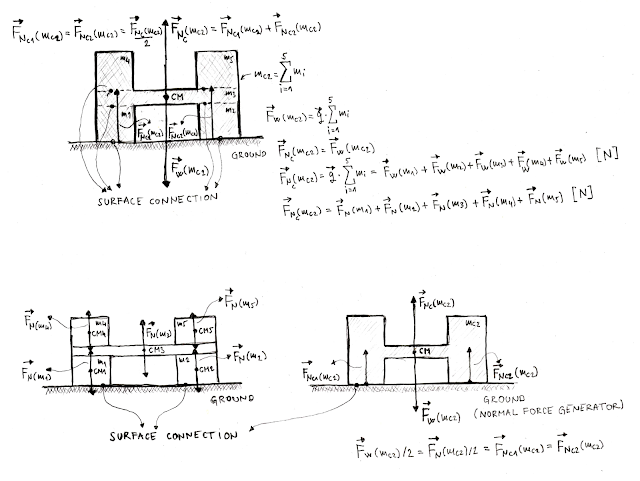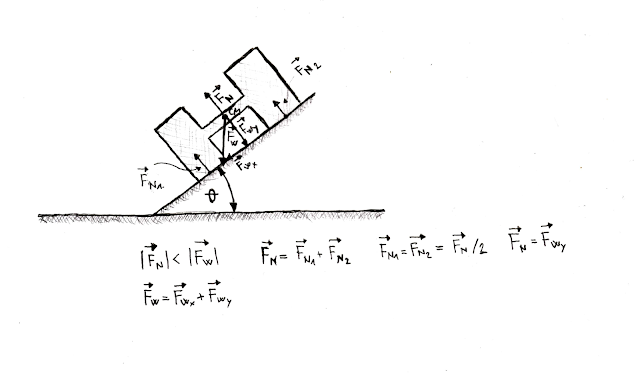### Feel the force around you – Normal force

This article is intended to explain the normal force as one of the parameters commonly used in the mobile robot hardware development. The normal force is the reactional force generated by the ground and has composite nature. It is very important to know something about normal force or better to understand its nature, if you are a person who like to build mobile robots from scratch. Normal force plays an important role regarding DC motor selection, because it is a frictional force actually, when it is multiplied by frictional coefficient. To explain the composite nature of the normal force we will see a couple of examples.Figure #1

What we have here are two masses m1 and m2 with the center of the mass CM1 and CM2, respectively. It is a starting point for our discussion. These two masses interface with the ground at the point marked as surface connection. On the right side of the Figure #1, two different forces were presented Fw and FN. FW is the weight (force) of the mass, while FN is the normal force generated by the ground and acts in the center of the mass, not at the point marked as surface connection (connection between mass and the ground). These two forces, in this case, are in equilibrium because of the same magnitude and opposite directions. The normal force is always directed upward from the surface (ground in this case) while weight force is always directed to the ground.Figure #2

Now, let’s place mass m3 to stand on masses m1 and m2 respectively, as it is shown on Figure #2 (left). Now, we do not have one surface connection, instead, two additional connections are presented. One surface connection stands between ground and masses m1 and m2. Second surface connection stands between masses m1 and m3 while third connection stand between masses m2 and m3. Now we can conclude the following: ground generates normal forces against masses m1 and m2, while masses m1 and m2 generate normal forces against mass m3, so that we have four normal forces in the summary. That is correct but not 100%. Do we really have four normal forces? No, we do not have, we have only one normal force. To explain only one normal force we have to think differently. Figure #2 shows three different masses: m1, m2 and m3, but actually there is only one mass, marked as mc1, as it is shown on Figure #2 (right). Mass mc1 is a composite mass, composed of masses: m1, m2 and m3, with only one center of the mass CM, with only one weight force and only one normal force generated by the ground (normal force generator). From the equations shown in the Figure #2 (right), you can see that normal force FNC(mc1) is composite force, composed of two normal forces: FNC1(mc1) and FNC2(mc1) generated by the ground respectively. Regarding magnitude, FNC1(mc1) and FNC2(mc1) are half smaller than FNC(mc1) normal force. On the other side, forces: FNC(mc1) and FW(mc1) are in equilibrium, because of the same magnitude and opposite directions, where both forces act on the center of the mass, marked as CM.Figure #3

Now, let’s place two additional masses: m4 and m5 to stand on mass m3. The number of surface connections are increased for two, so there are five surface connections in total. Two additional connections stand between masses m3 and m4 and masses m3 and m5. Mass m3 generates normal forces against masses m4 and m5. At this point, five normal forces are generated in summary, but as we already said, there are no five masses and five normal forces, there is only one mass, marked as mc2 and there is only one normal force FNC(mc2), generated by the ground surface, as it is shown on Figures #3. As we already have had a discussion, previously in this article, mass mc2 is composite mass, composed of masses: m1,, m5. The same stand for normal force FNC(mc2), it is a composite normal force, composed of normal forces: FN(m1), FN(m2), FN(m3), FN(m4) and FN(m5). As for the other examples, FNC(mc2) and FW(mc2) are equal forces (in equilibrium) regarding the magnitude, with opposite directions acting on the center of the mass, CM. The conclusion is simple: there is only one weight force (not kilograms ;-)), only one normal force, and it is always generated by the ground surface.Figure #4

In reality we do have different scenario, but not regarding normal force. Even if the mass could be deformed by applying external force, like applying weight of the mass m4, everything stand as it is regarding normal force. As there is only one mass, composite mass, even if the mass is deformed, there is only one weight force, only one normal force and only one center of the mass. If mass m3 is broken, by applying to large weight force m4, normal force will be divided into several different forces to support newly created composite masses. Also, it is very important to note here, that mass m1, for example, is also a composite mass, composed of the mass of the atoms.Figure #5

The normal force is not always in equilibrium with weight force. For inclines, the normal force is less regarding magnitude. In this case, the normal force is in equilibrium with only one component of the weight, since weight is also a composite force like normal force is. Inclines will be covered in separated article, because its play important role regarding frictional force and DC motor selection. At the end of this article we can say only this: feel the force around you!

Related articles:
Feel the force around you – Frictional force Part I

Feel the force around you – Frictional force Part III
Feel the force around you – Frictional force Part IV

Real catalog examples and calculations

1.What do you think, is it a normal force constant or not?

1.